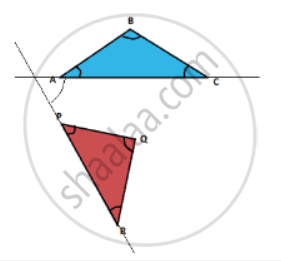# If you need to construct a triangle with point P as one of its vertices, which is the angle that you need to construct a side of the triangle? - Mathematics

MCQ

If you need to construct a triangle with point P as one of its vertices, which is the angle that you need to construct a side of the triangle?#### Options

• ∠QPR

• ∠RQP

• ∠PRQ

• Angle PR makes with AC

#### Solution

Angle PR makes with AC

Explanation:-

Once we know the position of Point P, we need to find the orientation of the ΔPQR w.r.t ΔABC. For that purpose, we need to know the angle of any one side that makes with the original triangle. Therefore, among the given options we will be able to determine the orientation of the ΔPQR if we know the angle which side PR makes with side AC.

Concept: Division of a Line Segment
Is there an error in this question or solution?